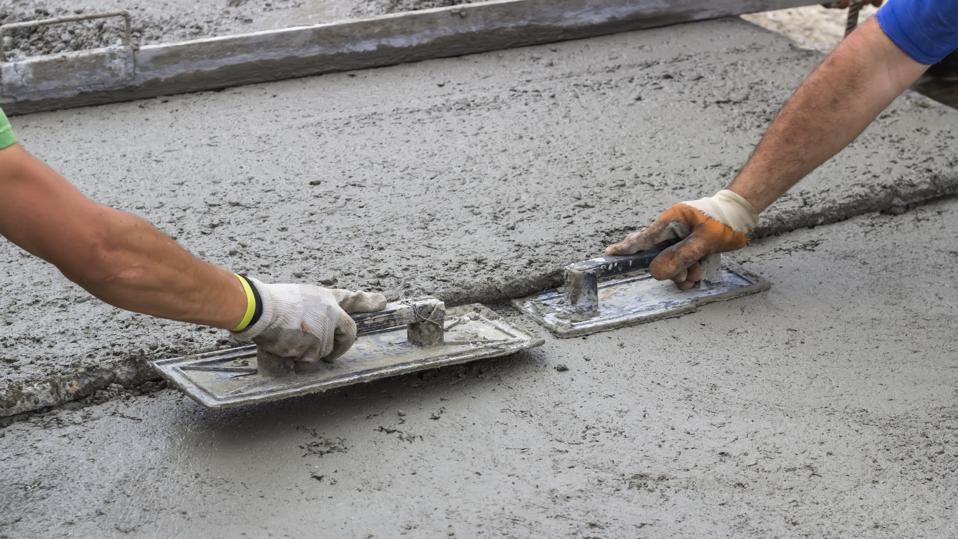Cement is a fast-setting and hardening binding material. It is created by combining lime or limestone with sand, clay, and other ingredients.

Among all the types of Cement, Concrete is one of the most common materials used globally in construction. Fine and coarse aggregate are bound together in a fluid cement (cement paste) that hardens over time. The aggregates are typically sand and gravel (or crushed stone), while the paste is composed of water and Portland cement. The cement and water harden and bind the aggregates into a rock-like mass through a process known as hydration. After 28 days, concrete has reached approximately 99 percent of its maximum compressive strength. This hardening process, however, can last for years, resulting in stronger concrete over time.

How do we calculate concrete?

Here's an example calculation:

Assume we have four concrete elements, each three feet long, three feet wide, and three feet tall. Now we multiply 4 times (3*3*3). Pre-mixed concrete is typically sold by the cubic yard, and this is the standard unit of measurement. Because construction elements are typically measured in feet, our calculator converts the volume to cubic yards - in our example, 4 cu yd (108 cubic feet).

Sometimes you'll know exactly how many slabs you need. Other times, you'll just know the area that needs to be paved - feel free to provide any of these values. In our example, we are paving 36 square feet.

After having the above measurements, we can now go to the essence of the matter.

1. Check to see if the density of a specific producer's concrete is the same as the default value in our calculator (150 lb/cu ft). Feel free to modify it as necessary.
2. The total weight of concrete required is now displayed.
3. Check to see if the weight of a single bag of cement is the same as the default value (60 lb). If not, change it to the appropriate number once more.
4. Be sure to account for spills and waste. Experienced contractors typically assume 5-10%.

Let's keep the default measures in our example. We require 4 cubic yards of cement, and our supplier sells it in 60-pound bags with a density of 150 pounds per cubic foot. The total amount of concrete required is 16,201.03 lb. Assuming a 5% loss due to waste and spillage - voila! 284 bags of concrete are required.

How would you know the cost of a Concrete Slab?

Once you've determined how much concrete you'll require (in bags) and how many slabs you'll need, simply enter the price of the bag to receive the concrete slab cost, the cost to pave your desired area, a cost per unit of volume, and the total cost of the material you require.

Reference: CEMEX - Ready-Mix Concrete Calculator - a handy calculator tool for check concrete cost in Philippines

• concrete cost per meter m2
• concrete cost per cubic meter m3
• concrete cost per yard delivered
• concrete cost per square foot

Shop at Buildeee

> Republic Cement (₱240)

> Bagger Concrete Mixer (₱26,000)

> Cement Trowel (₱ 55)

Planning to build a home? Buildeee Team will definitely help you with that. You can reach us through:

Viber/WeChat: 0917-109-3382